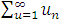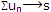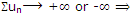# Describe the sum of a series

Publish On: 2019-03-18

Total Post: 559

# Mjay Jollay

Total Post: 0

## ANS: Describe the sum of a series

If a sequence <Sn>, of the partial sums of a series Σun converges to a finite quantity ‘s’, then ‘s’ is called the sum of the given series Σun and is generally written as    s == u1 + u2 + …. + un + ….     or simply as.

Remark 1:the series Σun diverges to +∞ (plus infinity) or to -∞ (minus infinity).

Remark 2: We know that the convergence of a sequence always depends upon from and after some fixed terms, therefore, the nature of the series remains unaltered if a finite number of terms are taken out from the series.

The following theorems are the general convention for converges or divergence of a series.

Theorem 1: The replacement, addition or omission of a finite number of terms of a series Σun has no effect on its convergence.

Theorem 2: The convergence of a series remains unchanged if each of its terms is multiplied by a constant by a constant k, k ≠ 0.